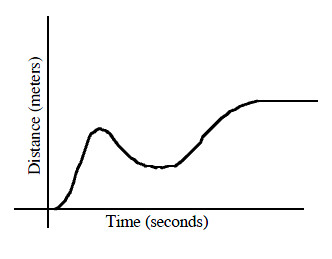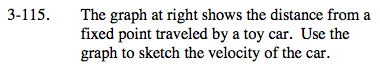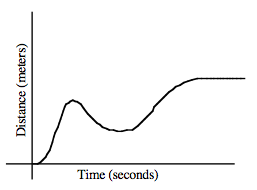### Home > CALC > Chapter 3 > Lesson 3.3.3 > Problem3-115

3-115.
1. The graph below shows the distance from a fixed point traveled by a toy car. Use the graph to sketch the velocity of the car. Homework Help ✎Notice that shortly into the car's journey, it changed directions and started moving back towards its starting position. How will this be reflected on a velocity graph?

A steep negative slope on a distance graph will be plotted as a very low y-value on a velocity graph. A steep positive slope on a distance graph will be plotted as a very high y-value on a velocity graph. No slope on a distance graph will be plotted on the x-axis of the velocity graph.